# Derivative of inverse secant

## Homework Statement

The two graphs are possible legitimate representations of ##y=\sec^{-1}(x)##.
The derivative is positive on all the domain and so is graph A, but graph B has negative tangent when x<-1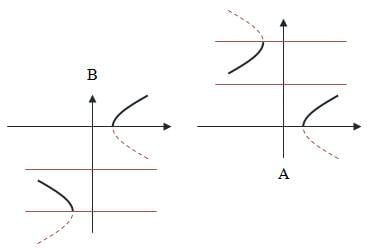## Homework Equations

Derivative of inverse secant:
$$(\sec^{-1}(x))'=\frac{1}{x(\pm\sqrt{x^2-1})}$$

## The Attempt at a Solution

The derivative was established on all the domain, so i think it must be positive. the slope must be pointing upwards on all the domain.
I don't know.

BvU
Homework Helper
Check the domain. figure B would be somewhat impractical.

figure B would be somewhat impractical.
Why? there is a 1 to 1 matching between x and y values. the picture is from a text book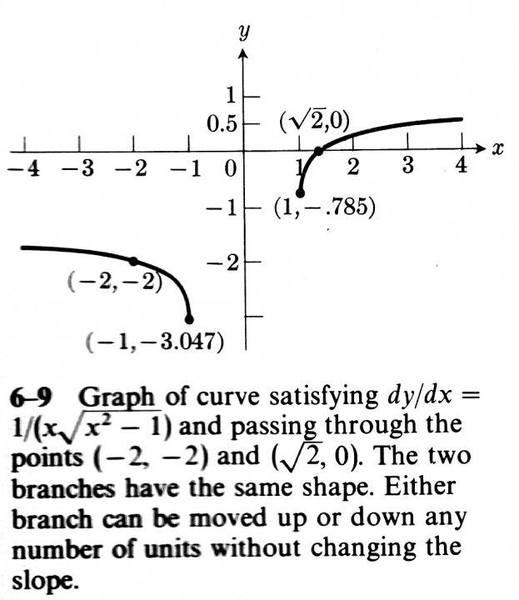BvU
Homework Helper
Since cos(x) is 1 to 1 on ##\ [0,\pi]\ ## I would expect the##\ \operatorname{asec}^{-1}\ ## to have the range ##\ [0,\pi]\ ## on the domain ##<-\infty,-1]\ \cup \ [1,\infty>\ ##.

Your derivative is correct only for ##x>1##.

Your derivative is correct only for ##\ x>1 \#.
For all x:
$$[\sec^{-1}(x)]'=\frac{1}{\lvert x \rvert \sqrt{x^2-1}}$$
Graph B is possible since: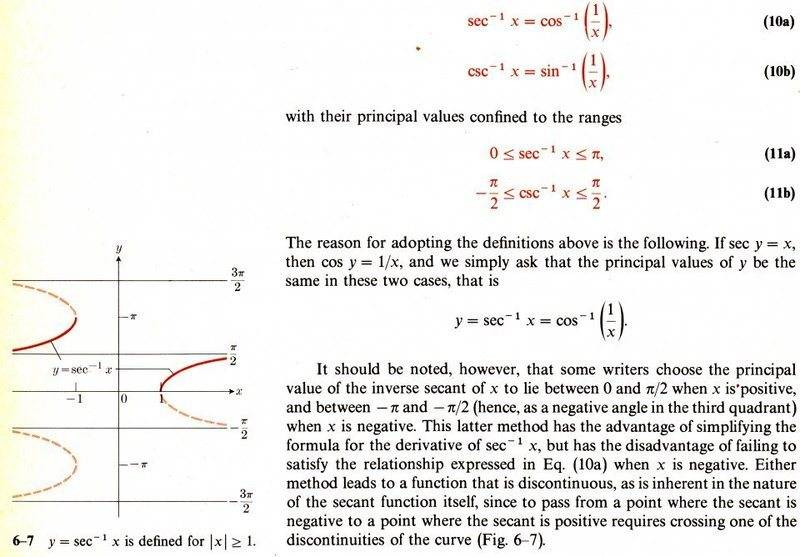I think they have a mistake, i don't see why "the later method has the disadvantage of failing to satisfy Eq. (10) when x is negative".
(10a) merely says:
$$y=\sec^{-1}(x)=\cos^{-1}\left( \frac{1}{x} \right)~\Rightarrow~x=\frac{1}{\cos(y)}$$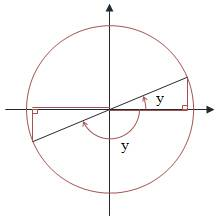when -π<y<-π/2, meaning going clockwise and in the third quadrant, x<0, so ## x=\frac{1}{\cos(y)}## is true, so figure B is also correct.

Last edited:
vela
Staff Emeritus
Homework Helper
It's because, by definition, ##0 \le \cos^{-1} x \le \pi##. If ##\sec^{-1} x < 0##, then it can't possibly equal ##\cos^{-1} \frac 1x## which is non-negative.

It's only by definition if that's how you choose to define it. Plenty of old books define it differently so as to avoid the whole issue of artificially inserting the absolute value.

vela
Staff Emeritus
Homework Helper
It's only by definition if that's how you choose to define it. Plenty of old books define it differently so as to avoid the whole issue of artificially inserting the absolute value.
So what? Karol's question was about what the book claimed, and the book uses the standard definition of inverse cosine.

So what? Karol's question was about what the book claimed, and the book uses the standard definition of inverse cosine.

I'm not sure what you mean by "so what?" His question was not about the arccosine, it was about the arcsecant and there seems to be a notion in the responses that there is a correct way to define it, despite the fact that Karol posted an image with an alternative definition. There is no relationship between the choices of restriction for the arccosine and arcsecant. There are an infinite number of ways to define any of the six inverse trig functions. The way that it is usually defined in modern textbooks requires the artificial introduction of an absolute value in the derivation of the derivative of the arcsecant and it appears that that was the point of his original question. If you define it differently, which is perfectly arbitrary, you can avoid the entire issue of the absolute value if the derivative is negative for negative arguments and positive for positive arguments. If you choose the restriction such that the derivative is positive for both positive and negative values of the argument then you have to artificially introduce the absolute value and I have to spend 15 minutes of lecture explaining this every time I teach calc 1.

vela
Staff Emeritus
Homework Helper
He wrote, "I don't see why 'the later method has the disadvantage of failing to satisfy Eq. (10) when x is negative'." His question seems pretty clear to me.

He wrote, "I don't see why 'the later method has the disadvantage of failing to satisfy Eq. (10) when x is negative'." His question seems pretty clear to me.

But that wasn't his original question which, to me, still appears unanswered.

i don't understand why:
If ##\sec^{-1} x < 0##, then it can't possibly equal ##\cos^{-1} \frac 1x## which is non-negative.
What's the difference between ##~\sec^{-1}(x)~## and ##~\cos^{-1} \left( \frac 1x \right)##?
$$y=\sec^{-1}(x)~\Rightarrow~\sec(y)=\frac {1}{\cos(y)}=x,~~y=\cos^{-1} \left( \frac 1x \right)~\Rightarrow~\cos(y)=\frac {1}{x}$$
They are the same, and for:
$$y=\sec^{-1}(x)<0~\Rightarrow~-\frac{\pi}{2}<y<-\pi$$
If x<0, as the book claims there's a problem:
$$y=\cos^{-1} \left( \frac 1x \right)~\Rightarrow~\cos(y)=\frac 1x~\Rightarrow~\cos(y)<0~\Rightarrow~-\frac{\pi}{2}<y<-\pi$$
No contradiction, the y's are the same

vela
Staff Emeritus
Homework Helper
The statements ##y=\cos x## and ##x = \cos^{-1} y## are not equivalent. When you define a function, you always have to specify its domain and codomain. You're ignoring this fact, which is leading to your confusion.

The cosine function maps a real number to a real number in the interval [-1,1]. According to the conventional definition, the inverse cosine function maps [-1,1] to ##[0,\pi]##. If you happen to have an angle between 0 and ##\pi##, then it's true that ##\cos^{-1} \cos x = x##, but that relationship does not generally hold. For example, say ##x = 2\pi##. Then you have ##\cos x = 1## but ##\cos^{-1} 1 = 0 \ne x##. It's not correct to say ##\cos^{-1} 1 = 2\pi## because ##2\pi## isn't in the codomain of the inverse cosine function.

Of course, you can, as Alan noted, define the inverse cosine function differently so that ##\cos^{-1} 1 = 2\pi##, but then you can't say that ##\cos^{-1} 1 = 0##.

The statements ##y=\cos x## and ##x = \cos^{-1} y## are not equivalent. When you define a function, you always have to specify its domain and codomain. You're ignoring this fact, which is leading to your confusion.

The cosine function maps a real number to a real number in the interval [-1,1]. According to the conventional definition, the inverse cosine function maps [-1,1] to ##[0,\pi]##. If you happen to have an angle between 0 and ##\pi##, then it's true that ##\cos^{-1} \cos x = x##, but that relationship does not generally hold. For example, say ##x = 2\pi##. Then you have ##\cos x = 1## but ##\cos^{-1} 1 = 0 \ne x##. It's not correct to say ##\cos^{-1} 1 = 2\pi## because ##2\pi## isn't in the codomain of the inverse cosine function.
Of course, you can, as Alan noted, define the inverse cosine function differently so that ##\cos^{-1} 1 = 2\pi##, but then you can't say that ##\cos^{-1} 1 = 0##.
But how does it answer the question with what ##~\sec^{-1}(x)~## differss from ##~\cos^{-1} \left( \frac 1x \right)~## when x<0?
If it's about the codomain, then according to the pic in post #5 ##~\sec^{-1}(x)~## is between [o,π], as is ##~\cos^{-1} \left( \frac 1x \right)~##
And another thing. the absolute value in the derivative of ##~\sec^{-1}(x)~## isn't related to choice of codomain: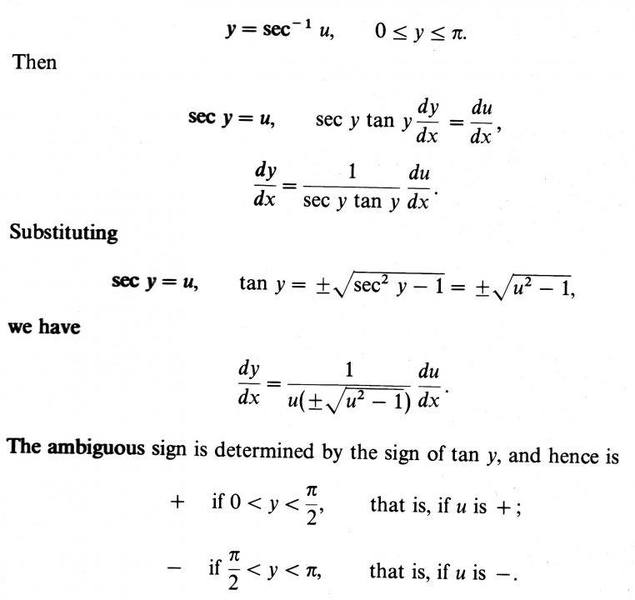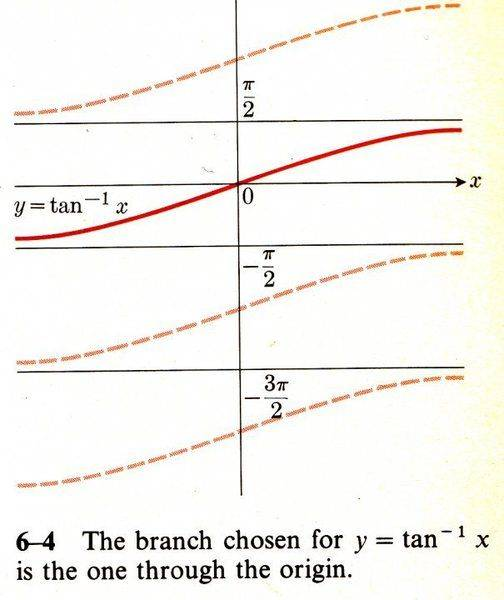vela
Staff Emeritus
Homework Helper
If it's about the codomain, then according to the pic in post #5 ##~\sec^{-1}(x)~## is between [o,π], as is ##~\cos^{-1} \left( \frac 1x \right)~##
I don't know if you're trying to be obtuse or what. You asked about the following excerpt: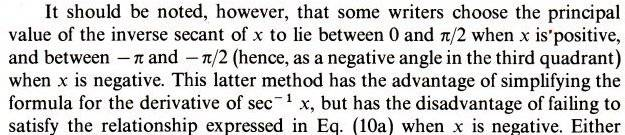It clearly says the codomain of the alternate ##\sec^{-1} x## is NOT ##[0,\pi]##.

It clearly says the codomain of the alternate ##\sec^{-1} x## is NOT ##[0,\pi]##.
So ##~\sec^{-1}(x)\neq \cos^{-1}\left( \frac1x \right)## when x<0 and when ##~\sec^{-1}(x)~##'s codomain is between ##[-\pi,-\frac{\pi}{2}]## because ##~\cos^{-1}\left( \frac1x \right)##'s codomain is ##[0,\pi]##?
And why, when ##~\sec^{-1}(x)~##'s codomain is between ##[-\pi,-\frac{\pi}{2}]##, it has an advantage of simplifying the derivative of ##~\sec^{-1}(x)~##?
The derivative, as i showed in post #14, doesn't depend on anything and is always positive?

SammyS
Staff Emeritus
Homework Helper
Gold Member
So ##~\sec^{-1}(x)\neq \cos^{-1}\left( \frac1x \right)## when x<0 and when ##~\sec^{-1}(x)~##'s codomain is between ##[-\pi,-\frac{\pi}{2}]## because ##~\cos^{-1}\left( \frac1x \right)##'s codomain is ##[0,\pi]##?
To clarify your statement regarding the alternate definition of the sec-1 function, which has a codomain including the interval [-π, -π/2 ):
##\displaystyle \sec^{-1}(x)\neq \cos^{-1}\left( \frac1x \right) \ ## when ##\ x<0 \,,\ ## because in this case, ##\displaystyle\ -\pi \le \sec^{-1}(x) < -\frac{\pi}{2} \ ## while ##\displaystyle\ \frac{\pi}{2} < \cos^{-1}\left(\frac 1 x \right) < \pi \ ##
And why, when ##~\sec^{-1}(x)~##'s codomain is between ##[-\pi,-\frac{\pi}{2}]##, it has an advantage of simplifying the derivative of ##~\sec^{-1}(x)~##?
The derivative, as i showed in post #14, doesn't depend on anything and is always positive?
It seems that you have previously answered this in posts #1 and #5, if not elsewhere, however, the statement you have here is not correct.

The simplification to the derivative, of course, is that the absolute value is eliminated for the alternate definition, whereas for the definition with the standard codomain, the derivative is positive, regardless of the sign of x and this requires the use of the absolute value or some equivalent expression.
.

In the book: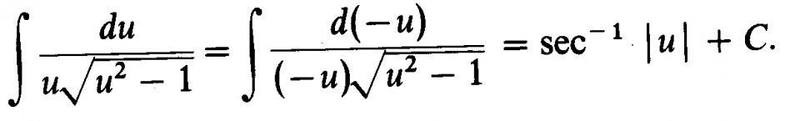I don't quite understand the d(-u), i think it says that the integral relates to the variable -u.
I guess the above line is because, for x>1:
$$\int \frac{dx}{x\sqrt{x^2-1}}=\sec^{-1}(x)$$
And for x<-1:
$$\int \frac{dx}{x\sqrt{x^2-1}}=\int \frac{d(-x)}{(-x)\sqrt{(-x)^2-1}} =\int \frac{d(-x)}{\lvert (-x)\rvert \sqrt{(-x)^2-1}} =\sec^{-1}(-x)$$
$$\left\{ \begin{array}{ll} \text{for}~x>1 & \sec^{-1}(x) \\ \text{for}~x<-1 &\sec^{-1}(-x) \end{array} \right.~\Rightarrow~\sec^{-1}\vert x \vert$$

I thank you all very much for your support
Karol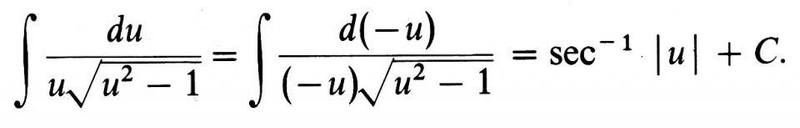What is the meaning of:
$$\int \frac{d(-x)}{(-x)\sqrt{x^2-1}}~?,~\int f(-x)d(-x)~?,~d(-x)?$$